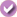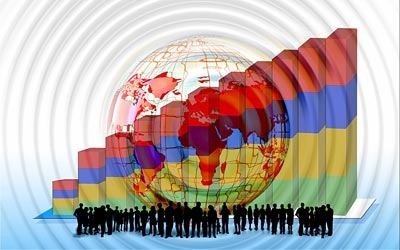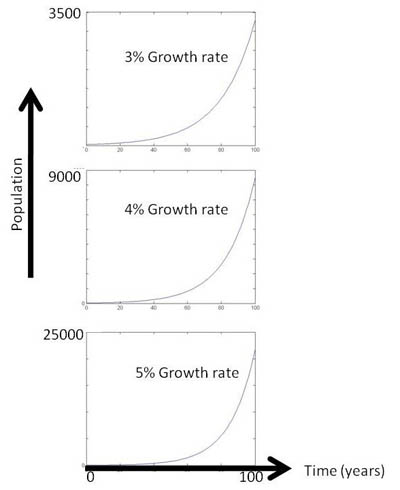# Crystal Ball Math: Predicting Population Growth with Models

Create Assignment

This feature requires that you be logged in as a Google Classroom teacher and that you have an active class in Google Classroom.

 Areas of Science Environmental Science Difficulty Time Required Average (6-10 days) Prerequisites Only arithmetic is needed to make the model; however, some exposure to simple programming will make this science fair project easier, and exposure to the Euler number, e, will make the model more understandable. Material Availability Readily available Cost Average (\$50 - \$100) Safety No issues

## Abstract

When is an animal considered endangered? When will it become extinct? What happens when a population has reached the limits of its resources? What happens to a population when a habitat changes? These are some of the questions population biologists try to answer. They use population models, created from math equations, to predict what will happen to a population over time. If you want to see how modeling is done and make some predictions of your own, you certainly won't need a crystal ball—just try this environmental science fair project!

## Objective

To learn some of the ways in which animal population growth is modeled, and then use the logistic model to determine how a population grows when it is far below, at, or far above its carrying capacity.

## Share your story with Science Buddies!Yes, I Did This Project! Please log in (or create a free account) to let us know how things went.

## Credits

Kristin Strong, Science Buddies

MATLAB® is a registered trademark of The MathWorks.

General citation information is provided here. Be sure to check the formatting, including capitalization, for the method you are using and update your citation, as needed.

### MLA Style

Science Buddies Staff. "Crystal Ball Math: Predicting Population Growth with Models." Science Buddies, 15 June 2018, https://www.sciencebuddies.org/science-fair-projects/project-ideas/EnvSci_p049/environmental-science/predicting-population-growth-with-models?class=AQVmaGCl4zpdHrRXzC59McDjEWbX1NLlXYeTpazEWHDdId4GHtv90vvtTl_qfBqm6gpn3An1hRv7zKBPsObafOPUFSMv-CMU9QZYdFrP4B__UA. Accessed 16 Dec. 2019.

### APA Style

Science Buddies Staff. (2018, June 15). Crystal Ball Math: Predicting Population Growth with Models. Retrieved from https://www.sciencebuddies.org/science-fair-projects/project-ideas/EnvSci_p049/environmental-science/predicting-population-growth-with-models?class=AQVmaGCl4zpdHrRXzC59McDjEWbX1NLlXYeTpazEWHDdId4GHtv90vvtTl_qfBqm6gpn3An1hRv7zKBPsObafOPUFSMv-CMU9QZYdFrP4B__UA

Last edit date: 2018-06-15

## IntroductionAnimal life is all around you, in beauty and abundance. Tiny microbes thrive on your healthy, normal skin; jellyfish float like lacy parachutes through the seas; red-faced turkey vultures cruise the skies, barely flapping at all as they scavenge for carcasses; and beady-eyed rodents scurry nervously through the fields. Such different-looking creatures, but they all have similar biological characteristics:

• They do not have rigid cell walls.
• They have a type of cell called eukaryotes.
• Their cells are specialized into different types of tissues, like skin and muscle.
• They try to keep their internal environments within a normal range (they use feedback control for homeostasis).
• They eat plants or animals to get the carbon and energy needed to survive (they are heterotrophs).
• Most of them move.
• All of them reproduce, usually with two sets of genetic material inside each of their cells.Figure 1. There are many types of animals, but they have similar biological characteristics. (Turkey vulture, Callie Bowdish, 2006; Rat, Kristin Kearns, 2009).

All animals also interact with their external environments, and their ability to grow and increase in numbers is limited by the amount of resources that are available to them. Resources include things they use to survive, things they eat and drink, and their habitat. Natural predators also influence an animal's numbers, or population. If an environment changes, due to human or natural factors, an animal's habitat and numbers of natural predators can be affected, and an animal's population can increase or decrease. An oil spill is a well-known example of how a human factor can impact the environment, affect a habitat, and change the populations of certain species. Another example in North America is the deer population. Many natural predators of deer—like wolves, mountain lions, and coyotes—have smaller populations than they did centuries ago, due to city growth and the resulting loss of predator habitats. Consequently, the deer population has increased.

Biologists sample and model populations to see if animals are present in healthy numbers, and if those numbers will remain healthy in the future. Modeling is a way of using math equations to predict what will happen to a population over time. The first and simplest model was developed in the late 1700's by Thomas Malthus. He noted that with unlimited resources, most populations will grow exponentially.

Equation 1:

 P(t) = P0ert
• P(t) is the population as a function of time, represented by a number.
• P0 is the initial population, represented by a number.
• e is Euler's number, which is approximately 2.718.
• r is the growth rate.
• t is time, often given in years.

For example, if you start with an initial population of 25 individuals, you can see in Figure 2 what will happen to the population in later years, at growth rates of 1 and 2 percent, if the animals have unlimited resources (unlimited habitat, unlimited food, and no predators). You can see from the curves that a 1 percent growth rate results in a doubling of the population about every 70 years. A 2 percent growth rates results in a doubling about every 35 years.Figure 2. These graphs show how a population will grow, according to the simple exponential model, over a 100-year time span, starting with an initial population of 25, and with growth rates of 1 and 2 percent.

If you look at even faster growth rates, like 3, 4, and 5 percent, you can see in Figure 3 how quickly the population grows, but the shape of all the curves is the same. These exponential J-curves have been said to hold true over the short term. However, in the real world, resources are generally limited, so over the long term, the Malthus model, which assumes unlimited resources, does not hold true.Figure 3. These graphs show how a population will grow, according to the simple exponential model (the Malthus model), over a 100-year time span, starting with an initial population of 25, and with growth rates of 3, 4, and 5 percent. Note that as time increases, all the curves begin to take on a "J" shape.

In the 1800's Pierre-François Verhulst tried to correct the model. He refined Malthus' model to include information about the carrying capacity, which is the size of a population that a habitat can support. The carrying capacity is the level at which the birth rate matches the death rate, resulting in a constant population over time. It is affected by factors such as food, number of predators, and competition for resources. Verhulst's model is given below.

Equation 2:

P(t) =
 P0ert K + P0 (ert - 1 )
• P(t) is the population as a function of time, represented by a number.
• P0 is the initial population, represented by a number.
• e is Euler's number, which is approximately 2.718.
• r is the growth rate.
• K is the carrying capacity.
• t is time, often given in years.

You can compare Equations 1 and 2 to see the different terms. Verhulst modified the Malthus model to show that if the starting population is well below the carrying capacity, K, the population will initially grow rapidly (like the J-curve), but the growth will slow as the population reaches its carrying capacity. This logistic model produces a population curve with an "S" shape, and so is called an S-curve. The model also predicts a different kind of growth behavior if the starting population is well above the carrying capacity. In this environmental science fair project, you will investigate what happens to a population when it is far below, at, or far above the carrying capacity of its habitat.

## Terms and Concepts

• Resource
• Predator
• Population
• Sample
• Model
• Exponential model
• J-curve
• Carrying capacity
• Logistic model
• S-curve
• Difference equation
• Differential equation
• Variable
• Initial
• For loop
• Static
• Time-varying
• Lotka-Volterra model
• Invasive species

### Questions

• What characteristics are the same in all animals?
• What factors affect an animal's population?
• Why is modeling useful?
• Based on your research, what is a Malthusian catastrophe?
• How does the Verhulst model differ from the Malthus model?

## Bibliography

This source describes the characteristics of all animals:

This source describes what makes populations grow and regulate themselves:

This source describes the simple, exponential (Malthus) model:

This source describes the logistic model:

This source discusses many types of population models:

This source describes difference equations:

This source discusses carrying capacity:

This source defines the human population growth rates of different countries:

This source shows you how population growth models can be used to predict the populations of elephants in Kruger National Park:

## News Feed on This Topic, ,
Note: A computerized matching algorithm suggests the above articles. It's not as smart as you are, and it may occasionally give humorous, ridiculous, or even annoying results! Learn more about the News Feed

## Materials and Equipment

Note: A programming tool, like a programmable calculator or a computer, will make the calculations in this experiment faster, but it is possible to do the experimental procedure with a simple calculator, or even pen and paper.

• Optional: Programmable calculator or computer with programmable software, like the student version of MATLAB®; available online (such as from www.mathworks.com/academia/student_version, or free to your teacher as an evaluation copy. For a student, the correct "Buy Now" link is in the right margin. You will need to prove your student status).
• Lab notebook
.Crystal Ball Math: Predicting Population Growth with Models

#### https://www.sciencebuddies.org/science-fair-projects/project-ideas/EnvSci_p049/environmental-science/predicting-population-growth-with-models

PDF date: 2019-12-16

## Experimental Procedure

Important Notes Before You Begin:

To experiment with the logistic model on a programmable calculator, computer, or in a table, you need to first convert the model into a difference equation. Equation 2, in the Introduction, is the solution to a first-order differential equation, and when that differential equation is converted to a difference equation, you get:

Equation 3:

 Population[time+1] = Population[time] + GrowthRate × ( 1 - Population[time] CarryingCapacity ) × Population[time]
• Population[time+1] is the population at the next time increment.
• Population[time] is the population at the current time increment.
• GrowthRate is the growth rate.
• CarryingCapacity is the carrying capacity.
• time is often given in years.

This difference equation is a good approximation to what is going on in the differential equation, and is in a form that can be readily implemented on a programmable tool, like a computer or calculator.

### Implementing Equation 3

You can implement Equation 3 with a programmable tool or in a table.

If you choose to use a programmable tool, a sample program of how to implement Equation 3 in software, like MATLAB, is given below. Notice that variables—like the growth rate, carrying capacity, initial population, population array, fraction of the carrying capacity array, and number of years to be evaluated—are defined and given initial (starting) values. Note: For your science fair project, you will experiment with changes to the initial values for the growth rate, carrying capacity, initial population, and number of years to be evaluated.

Following the initialization of the variables, a for loop is used to pass, in yearly steps, through time. Finally, results are plotted.

clear
growthrate=0.15;
carryingcapacity=400;
initialpop=30;
population(1)=initialpop;
numberofyears=50;
for time=1:numberofyears,
fractioncc(time)=population(time)/carryingcapacity;
population(time+1)=population(time)+growthrate*(1-fractioncc(time))*population(time);
end
fractioncc(time+1)=population(time+1)/carryingcapacity;
figure
plot(0:numberofyears,fractioncc)
figure
plot(0:numberofyears,population)

If you choose to use a table and pen and paper, create a table, so that you can see what the value of each of the variables will be with each time step. You will step through the table, just as the computer steps, in yearly increments, through the "for loop."

### Evaluating Equation 3

After implementing Equation 3 on a programmable tool, or in a table, you are ready to begin evaluating Equation 3 and testing the logistic population model.

1. Select a growth rate, carrying capacity, and the number of years to evaluate. You can pick any growth rate, carrying capacity, and time span that interests you, or you can do research on an animal that interests you to get its numbers. For example, states in the United States have a Department of Natural Resources website where you can get numbers on the current carrying capacity and growth rates of some species for that state. Remember that the carrying capacity and growth rate (sometimes called the intrinsic population growth rate or lambda) are not static (fixed) numbers. They depend upon the location, and change over time due to changes in habitat, predation, and competition from other species. Here are some representative numbers that you may choose to investigate:

 Animal Carrying capacity Growth rate Gray wolf 300–500 (Wisconsin) 21% Moose 840 (Quebec boreal forest) 25% Woodland caribou 200 (Quebec boreal forest) 24.5% Elephant 7,500 (Kruger National Park) 15%

1. Select three cases for an initial (starting) population:
1. Case 1: Make the initial population much less than the carrying capacity.
2. Case 2: Make the initial population equal to the carrying capacity.
3. Case 3: Make the initial population much more than the carrying capacity.
2. Make two line graphs for each case. First, plot the fraction of the carrying capacity on the y-axis and the time (in years) on the x-axis. Second, plot the population on the y-axis, and the time (in years) on the x-axis.
3. If desired, repeat steps 1–3 for a different growth rate and carrying capacity.

1. What was the shape of the curves for each of the cases? S-shape? J-shape? Backwards J-shape? In which case was there rapid growth in the population initially (like the Malthus model)? In which case was there rapid decline? Did the shape of the "fraction of the carrying capacity" curves match the population curves? What do you think would happen if you plotted the fraction of the carrying capacity on the x-axis and population on the y-axis? If you looked at different growth rates, did changing the growth rate change the shape of the curves?
.

## If you like this project, you might enjoy exploring these related careers:

### Mathematician

Mathematicians are part of an ancient tradition of searching for patterns, conjecturing, and figuring out truths based on rigorous deduction. Some mathematicians focus on purely theoretical problems, with no obvious or immediate applications, except to advance our understanding of mathematics, while others focus on applied mathematics, where they try to solve problems in economics, business, science, physics, or engineering. Read more

### Zoologist and Wildlife Biologist

Ever wondered what wild animals do all day, where a certain species lives, or how to make sure a species doesn't go extinct? Zoologists and wildlife biologists tackle all these questions. They study the behaviors and habitats of wild animals, while also working to maintain healthy populations, both in the wild and in captivity. Read more

### Environmental Scientist

Have you ever noticed that for people with asthma it can sometimes be especially hard to breathe in the middle of a busy city? One reason for this is the exhaust from vehicles. Cars, buses, and motorcycles add pollution to our air, which affects our health. But can pollution impact more than our health? Cutting down trees, or deforestation, can contribute to erosion, which carries off valuable topsoil. But can erosion alter more than the condition of the soil? How does an oil spill harm fish and aquatic plants? How does a population of animals interact with its environment? These are questions that environmental scientists study and try to find answers to. They conduct research or perform investigations to identify and eliminate the sources of pollution or hazards that damage either the environment or human and animal health. Environmental scientists are the stewards of our environment and are committed to keeping it safe for future generations. Read more

## Variations

• In the logistic model given above, the carrying capacity is static—it does not change with time. Investigate what happens when the carrying capacity is time-varying. A time-varying carrying capacity can model what happens seasonally to populations (due to weather changes).
• The Lotka-Volterra equations are a pair of differential equations used to model interactions between two species, like a predator and prey. Implement the predator-prey equations in difference-equation form on a programmable tool and investigate what happens to two populations simultaneously. Do the peaks and valleys in the populations occur at the same time? Or are they shifted apart from each other in time? If so, by how much are they shifted? Use classic population studies of wolves and moose, done at places like Isle Royale National Park in Lake Superior, to help you in your experiment.
• Invasive species, such as zebra mussels in the Great Lakes, have caused extensive ecological damage. Investigate, through a model, how an invasive species grows.
• Investigate, through a model, how habitat loss affects the polar bear population.
• Compare the population growths of a K-selected and an R-selected species.

## Share your story with Science Buddies!Yes, I Did This Project! Please log in (or create a free account) to let us know how things went.

The Ask an Expert Forum is intended to be a place where students can go to find answers to science questions that they have been unable to find using other resources. If you have specific questions about your science fair project or science fair, our team of volunteer scientists can help. Our Experts won't do the work for you, but they will make suggestions, offer guidance, and help you troubleshoot.